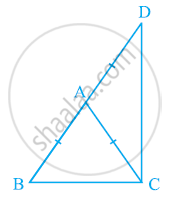# ΔABC is an isosceles triangle in which AB = AC. Side BA is produced to D such that AD = AB (see the given figure). Show that ∠BCD is a right angle. - Mathematics

ΔABC is an isosceles triangle in which AB = AC. Side BA is produced to D such that AD = AB (see the given figure). Show that ∠BCD is a right angle.#### Solution

In ΔABC,

AB = AC (Given)

⇒ ∠ACB = ∠ABC (Angles opposite to equal sides of a triangle are also equal)

In ΔACD,

⇒ ∠ADC = ∠ACD (Angles opposite to equal sides of a triangle are also equal)

In ΔBCD,

∠ABC + ∠BCD + ∠ADC = 180º (Angle sum property of a triangle)

⇒ ∠ACB + ∠ACB +∠ACD + ∠ACD = 180º

⇒ 2(∠ACB + ∠ACD) = 180º

⇒ 2(∠BCD) = 180º

⇒ ∠BCD = 90º

Concept: Properties of a Triangle
Is there an error in this question or solution?
Chapter 7: Triangles - Exercise 7.2 [Page 124]

#### APPEARS IN

NCERT Class 9 Maths
Chapter 7 Triangles
Exercise 7.2 | Q 6 | Page 124

Share